## 求一个PHP正则表达式把html中的所有标签属性全部去掉

```//这个地方不知道怎么写，过滤所有标签的属性，除img的src和alt
\$html=preg_replace("/<([a-zA-Z]+)[^>]*>/","<\\1>",\$html);```

```<table......属性一大堆>
<tr...属性一大堆><td>1.</td><td><span>随便一些字</span></td></td>
<tr...属性一大堆><td>2.</td><td><span>随便一些字</span></td></td>
</table>```

```<p>
1.<span>随便一些字</span>
2.<span>随便一些字</span>
</p>```

0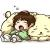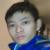strip_tags这个方法是可以，但会全部干掉，但是我要保留img标签怎么破，还有a标签的title属性之类的
00#### 引用来自“Liuxd”的评论

https://github.com/liuxd/CodeSnippets/blob/master/PHP/XssFilter.php

```<?php
/**
* Prevent XSS.
* Allowing you to add permmited tags or attributes.
*
* @author liuxd
*/
//error_reporting(E_ALL && ~E_NOTICE);
class XssFilter
{
/**
* Set white list.
* @param array \$p_aTags The tags you need.
* @param array \$p_aAttr The attributes you need.
*/
public function __construct(\$p_aTags, \$p_aAttr)
{
\$this->aTags = \$p_aTags;
\$this->aAttr = \$p_aAttr;
}
/**
* Process the String.
* @param string \$p_sInput
* @return string
*/
public function process(\$p_sInput)
{
list (\$bReturn, \$sInput) = \$this->filter(\$p_sInput);
if (!\$bReturn) {
\$sInput = \$this->process(\$sInput);
}
return \$sInput;
}
/**
* Filter.
* @param string \$p_sInput
* @return array
*/
private function filter(\$p_sInput){
\$aMatches = array();
\$sPattern = '/<([a-zA-Z0-9]+).*?>.*?<\/\1>/';
preg_match_all(\$sPattern, \$p_sInput, \$aMatches);
\$sInput = \$p_sInput;
\$bReturn = true;
if (!empty(\$aMatches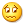)) {
list (\$aContents, \$aTags) = \$aMatches;
foreach (\$aTags as \$iKey => \$sTag) {
if (!in_array(\$sTag, \$this->aTags)) {
\$bReturn = false;
\$sInput = str_replace(\$aContents[\$iKey], '', \$sInput);
} else {
\$aTag = array();
preg_match('/<' . \$sTag . '.*?>/', \$aContents[\$iKey], \$aTag);
\$aAttr = explode(' ', \$aTag);
if (!\$this->checkAttr(\$aAttr)) {
\$bReturn = false;
\$sInput = str_replace(\$aContents[\$iKey], '', \$sInput);
}
}
}
}
return array(\$bReturn, trim(\$sInput));
}
/**
* Check tags' attributes.
* @param array \$p_aAttr
* @return bool
*/
private function checkAttr(\$p_aAttr)
{
\$bResult = true;
foreach (\$p_aAttr as \$sAttr) {
\$sAttrName = strstr(\$sAttr, '=', true);
if (\$sAttrName === false) {
continue;
}
if (!in_array(\$sAttrName, \$this->aAttr)) {
\$bResult = false;
}
}
return \$bResult;
}
}
\$obj = new XssFilter(array(), array('alt','title','src'));
\$str2 = '<h3><h1>dear</h1></<span>Hello</span>h3><div class="no">world</div><div id="ha">My name is</div>liuxd<img aid="4702" alt="大撒旦撒打算的" class="zoom" file="http://www.oschina.net/img/logo.svg?date=20151013" id="aimg_4702" initialized="true" inpost="1" src="http://www.oschina.net/img/logo.svg?date=20151013" style="word-wrap: break-word; cursor: pointer;" title="大撒旦撒打算的" width="600" zoomfile="http://www.oschina.net/img/logo.svg?date=20151013" />';
\$r2 = \$obj->process(\$str2);
echo \$r2;```

0```\$body = strip_tags(\$body,'<img><a>');
\$body = preg_replace("/<img(.*)src\s*=\s*\"([^\"]+)\"[^>]+>/isU","<img src='\\2' />",\$body);```

0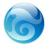htmlpurifier.org 我们的蝉知建站系统的html标准化使用的就是这套类库。功能相当强大。0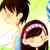```function rehtml(\$data){
\$data = preg_replace("/<em([^>]+)?>/i", "", \$data);
\$data = preg_replace("/<\/em>/i", "", \$data);
\$data = preg_replace("/<img([^>]+)?>/i", "", \$data);
\$data = preg_replace("/<div([^>]+)?>/i", "", \$data);
\$data = preg_replace("/<\/div>/i", "", \$data);
\$data = preg_replace("/<table([^>]+)?>/i", "", \$data);
\$data = preg_replace("/<\/table>/i", "", \$data);
\$data = preg_replace("/<tr([^>]+)?>/i", "", \$data);
\$data = preg_replace("/<\/tr>/i", "", \$data);
\$data = preg_replace("/<td([^>]+)?>/i", "", \$data);
\$data = preg_replace("/<\/td>/i", "", \$data);
\$data = preg_replace("/<tbody([^>]+)?>/i", "", \$data);
\$data = preg_replace("/<\/tbody>/i", "", \$data);
\$data = preg_replace("/<strong([^>]+)?>/i", "", \$data);
\$data = preg_replace("/<\/strong>/i", "", \$data);
\$data = preg_replace("/<p([^>]+)?>/i", "<p>", \$data);
\$data = preg_replace("/<span([^>]+)?>/i", "<p>", \$data);
\$data = preg_replace("/<\/span>/i", "</p>", \$data);
\$data = preg_replace("/<h1([^>]+)?>.*<\/h1>/i", "", \$data);
\$data = preg_replace("/<h2([^>]+)?>.*<\/h2>/i", "", \$data);
\$data = preg_replace("/<font([^>]+)?>/i", "", \$data);
\$data = preg_replace("/<\/font>/i", "", \$data);
\$data = preg_replace("/<center([^>]+)?>/i", "", \$data);
\$data = preg_replace("/<\/center>/i", "", \$data);
\$data = preg_replace("/<b ([^>]+)?>.*?<\/b>/i", "", \$data);
\$data = preg_replace("/<a [^>]*>.*?<\/a>/i", "", \$data);
return \$data;
}```